×
Get Full Access to Control Systems Engineering - 7 Edition - Chapter 3 - Problem 1
Get Full Access to Control Systems Engineering - 7 Edition - Chapter 3 - Problem 1

×

# Represent the electrical network shown in Figure P3.1 in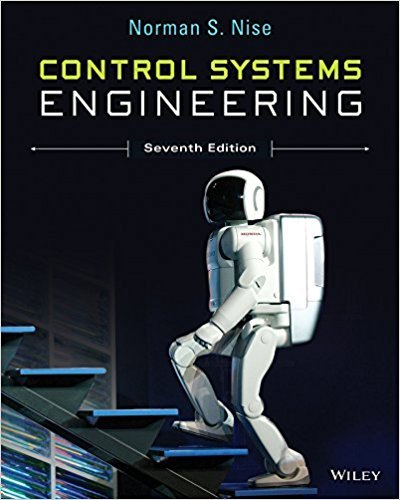ISBN: 9781118170519 162

## Solution for problem 1 Chapter 3

Control Systems Engineering | 7th Edition

• Textbook Solutions
• 2901 Step-by-step solutions solved by professors and subject experts
• Get 24/7 help from StudySoup virtual teaching assistantsControl Systems Engineering | 7th Edition

4 5 1 354 Reviews
24
3
Problem 1

Represent the electrical network shown in Figure P3.1 in state space, where vo(t) is the output. [Section: 3.4]

Step-by-Step Solution:

Problem 1

Represent the electrical network shown in Figure P3.1 in state space, where vo(t) is the output. [Section: 3.4]Step-by-step solution

Step 1 of 2

Add the branch currents and node voltages to the network.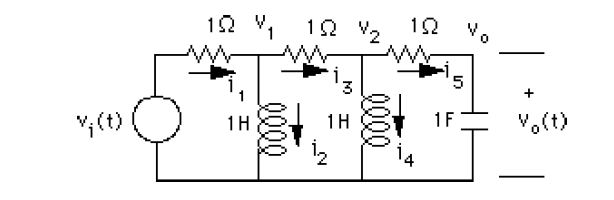Write the differential equation for each energy storage element,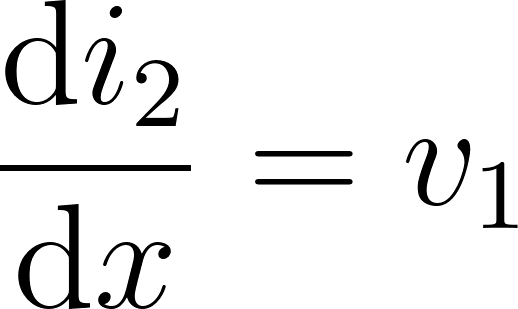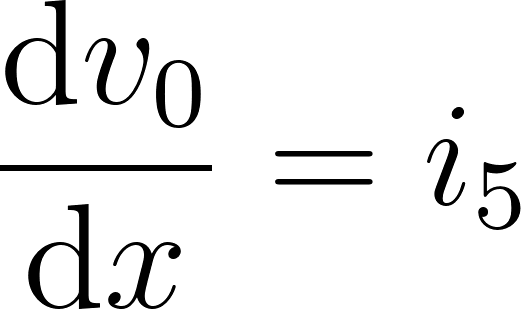Therefore,the state vector isNow obtain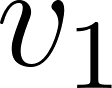,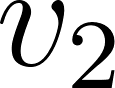and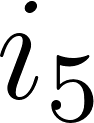in terms of the state variables.First find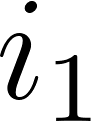in terms of the state variables.But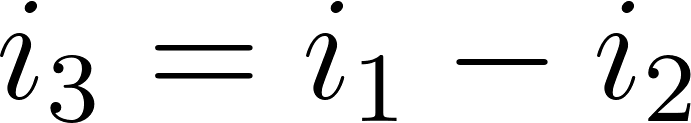andthus,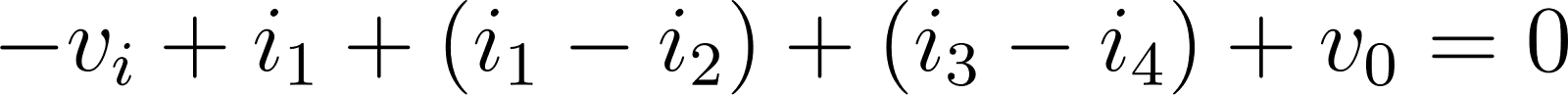Making substitution for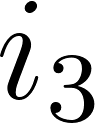yields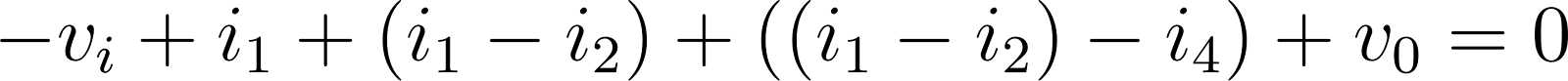Step 2 of 2

##### ISBN: 9781118170519

Unlock Textbook Solution